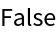Function Repository Resource:

ConnectedHypergraphQ

Determine whether a hypergraph is connected

Contributed by: Stephen Wolfram
 ResourceFunction["ConnectedHypergraphQ"][graph] yields True if graph corresponds to a connected hypergraph, and False otherwise.

Examples

Basic Examples (1)

Determine whether hypergraphs with ternary edges are connected:

 In:=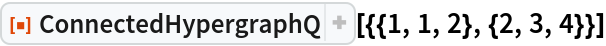Out=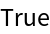In:=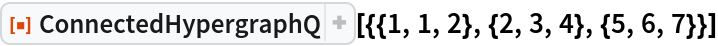Out=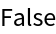Scope (1)

ConnectedHypergraphQ works with hyperedges of any length:

 In:=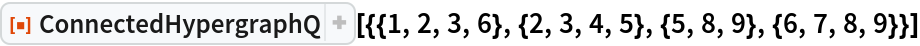Out=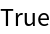In:=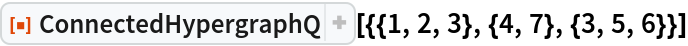Out=